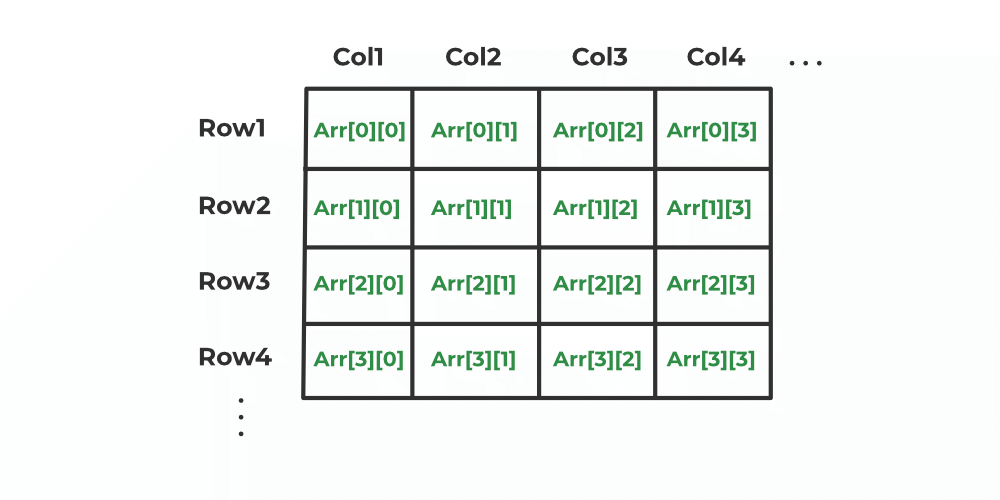Open in App
Not now

# C++ Return 2D Array From Function

• Last Updated : 22 Dec, 2022

An array is the collection of similar data-type stored in continuous memory. And when we are storing an array inside an array it is called 2 D array or 2-dimensional array. To know more about arrays refer to the article Array in C++.When there is a need to return a 2D array from a function it is always hard to find the way to do that, and the reason why it is hard because when we return an array local to some function it only returns the address of that array and once the function returning address is completed and we have reached to the place where we had called it. Then operating system will destroy all the memory which was created in that function so in that case the array that we get from that is also destroyed and we are holding the address of the illegal memory area.

So, in this article, we will see how to deal with this problem.

## Methods to Return 2D array From function

here, we will see returning of a 2D array from the function using the below methods:

• Using Dynamic Array
• Using Static Keyword
• Using Struct technique

### 1. Return 2D Array from Function in C++ Using Dynamic Array

Dynamic Array helps us to store arrays address using Pointer. So, we can use a dynamic array to use pointer-to-pointer notation to dynamically allocate and return an array. To know more about the dynamic array refer to Dynamic array in C++.

Below is the implementation of the above approach

## C++

 `// C++ code for returning 2D array``// from function using Dynamic array``#include ``using` `namespace` `std;` `const` `int` `N = 3;` `// function to display array``void` `printArray(``int``** arr)``{``    ``for` `(``int` `i = 0; i < N; ++i) {``        ``for` `(``int` `j = 0; j < N; ++j) {``            ``cout << arr[i][j]<<``" "``;``        ``}``        ``cout << endl;``    ``}``}` `// function to initialize and returning array``int``** getArray()``{``    ``int``** arr = ``new` `int``*[N];``    ``for` `(``int` `i = 0; i < N; ++i) {``        ``arr[i] = ``new` `int``[N];``        ``for` `(``int` `j = 0; j < N; ++j) {``            ``arr[i][j] = i + j;``        ``}``    ``}``    ``return` `arr;``}` `// Driver Code``int` `main()``{``    ``int``** arr;``    ``arr = getArray();``    ``printArray(arr);``    ``return` `0;``}`

Output

```0 1 2
1 2 3
2 3 4 ```

### 2. Return 2D Array from Function in C++ Using Static Keyword

The static keyword is used so to make the memory of the element static which means even when it is passed on the function it used the same memory element rather than making a copy of the element. To know more about static keywords refer to static keyword in C++.

Below is the implementation of the above approach

## C++

 `// C++ code for returning 2D array``// from function using static keyword``#include ``using` `namespace` `std;``const` `int` `N = 3;` `// function for display array``void` `printArray(``int` `arr[][N])``{``    ``for` `(``int` `i = 0; i < N; ++i) {``        ``for` `(``int` `j = 0; j < N; ++j) {``            ``cout << arr[i][j]<<``" "``;``        ``}``        ``cout << endl;``    ``}``}` `// function to initialize and returning array``int` `(*(getArray)())[N]``{``    ``static` `int` `arr[N][N]``        ``= { { 0, 1, 2 }, { 3, 4, 5 }, { 6, 7, 8 } };``    ``return` `arr;``}` `// Driver code``int` `main()``{``    ``int``(*arr)[N];``    ``arr = getArray();``    ``printArray(arr);``    ``return` `0;``}`

Output

```0 1 2
3 4 5
6 7 8 ```

### 3. Return 2D Array from Function in C++ Using Struct technique

Struct is the user-defined data type that can store multiple members bound together and acts like a single unit. So, we can use a struct having an array inside it as a unit to perform any operation and return it as a unit.

Below is the implementation of the above approach

## C++

 `// C++ code for returning 2D array``// from function using static keyword``#include ``using` `namespace` `std;``const` `int` `N = 3;` `// structure of array``struct` `ArrStruct {``    ``int` `arr[N][N];``};` `// function for display array``void` `printArray(ArrStruct var)``{``    ``for` `(``int` `i = 0; i < N; ++i) {``        ``for` `(``int` `j = 0; j < N; ++j) {``            ``cout << var.arr[i][j] << ``" "``;``        ``}``        ``cout << endl;``    ``}``}` `// function to initialize and returning array``ArrStruct getArray()``{``    ``ArrStruct var;``    ``for` `(``int` `i = 0; i < N; ++i) {``        ``for` `(``int` `j = 0; j < N; ++j) {``            ``var.arr[i][j] = i + j;``        ``}``    ``}``    ``return` `var;``}` `// Driver code``int` `main()``{``    ``ArrStruct arr;``    ``arr = getArray();``    ``printArray(arr);``    ``return` `0;``}`

Output

```0 1 2
1 2 3
2 3 4 ```

My Personal Notes arrow_drop_up